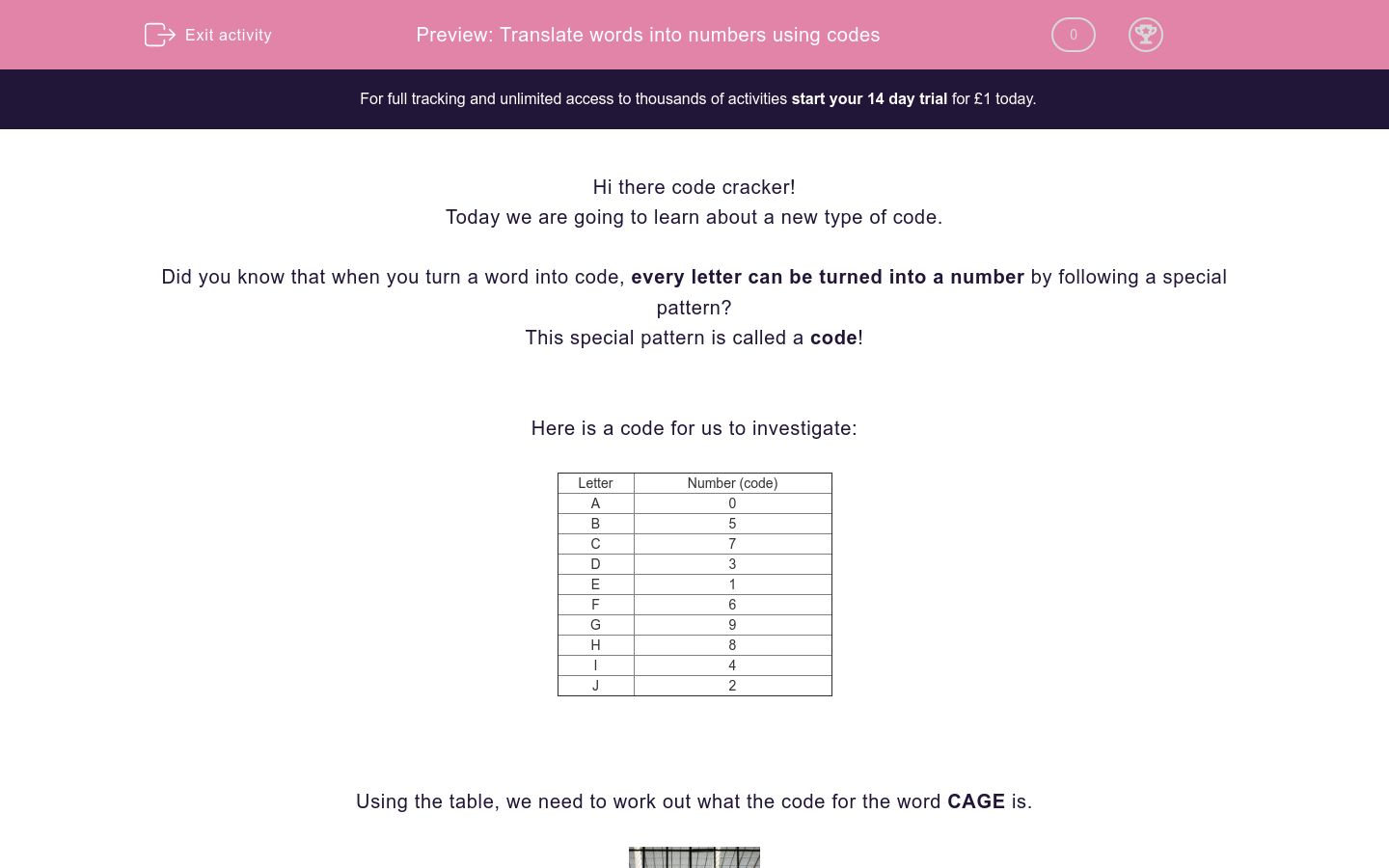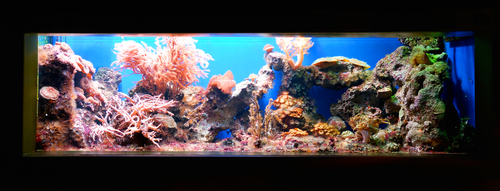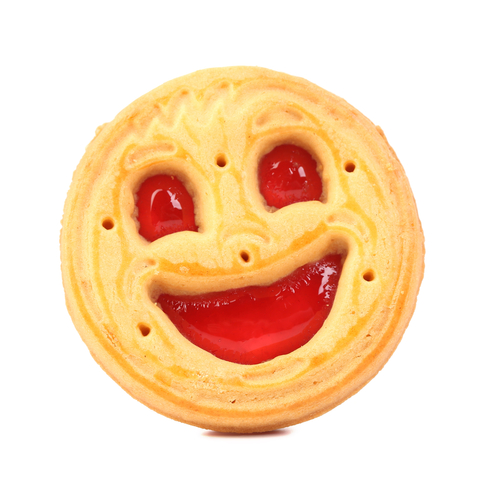# Translate words into numbers using codes

In this worksheet, students will turn words into number codes using a given code table as a base. It will develop their encoding and problem solving skills.Key stage:  KS 2

Curriculum topic:   Verbal Reasoning

Curriculum subtopic:   Number Code Breaking

Difficulty level:### QUESTION 1 of 10

Hi there code cracker!

Today we are going to learn about a new type of code.

Did you know that when you turn a word into code, every letter can be turned into a number by following a special pattern?

This special pattern is called a code!

Here is a code for us to investigate:

 Letter Number (code) A 0 B 5 C 7 D 3 E 1 F 6 G 9 H 8 I 4 J 2

Using the table, we need to work out what the code for the word CAGE is.If we start with finding the code for C and then work through each letter one-by-one, we find that the code for CAGE is 7091.

Now let’s reverse what we did to find out which word is represented by the code 8103.

Then we can repeat this process for each of the numbers one-by-one to find that the word is HEAD.

Is that what you found?

It’s now your turn to be a code cracker and change words into and out of code. Make sure you check the code table for each question.

Good luck code cracker!

Welcome to another number code cracking challenge, code cracker! I hope you're feeling energised and ready to go! You have made great progress so far.

 E = 1 G = 2 I = 5 L = 8 N = 7 O = 4 R = 3 S = 6 T = 9

We are going to the zoo today! Help me turn some of the animal names into number codes (and vice versa in some cases!).

The first animals we go to visit are the LIONS and TIGERS. What is the code for each animal?Write the codes into the correct boxes below.

 A = 2 B = 5 E = 6 F = 8 I = 0 L = 7 T = 1 U = 3

Right, then, let's keep going on our trip through the zoo!We now move on to the zebras. I love zebras! In fact, I would describe them as BEAUTIFUL animals. What is the code for the word BEAUTIFUL?

 A = 1 C = 3 L = 4 O = 9

OK, code cracker, on to another question! You are doing brilliantly so far!

So, I think it's time to stop for a drink. I'm getting thirsty!

I am going to choose a can of 3941. What am I going to choose to drink?A = 4 I = 1 M = 6 Q = 8 R = 0 U = 2

Now that we're feeling refreshed, I'm ready to see some more animals!

Now, let's head to the AQUARIUM.Can you work out the code for the word AQUARIUM. It's a long word, so make sure you write it out!

 C = 1 F = 2 H = 5 I = 8 L = 7 N = 4 O = 3 S = 6 W = 9

Great stuff, code cracker. Let's stay in the aquarium for now and look at some animals.My favourite fish is a CLOWNFISH, like the one above. What is the code for CLOWNFISH?

 A = 6 G = 9 I = 4 L = 5 O = 7 R = 2 S = 3

OK, code cracker, we are now over halfway. Brilliant work!So, let's move on to another animal enclosure. Now, I want to see the 97245563. Which animals do I want to see?

Giraffes

Monkeys

Gorillas

 A = 2 G = 7 N = 3 O = 6 R = 8 S = 4 T = 5 U = 1

This is great stuff, code cracker! You are doing brilliantly. Let's keep going - you only have a few questions left. Wahoo!

So, now we are moving on to look at the ORANGUTANS.What is the code for the word ORANGUTANS?

6823715234

6823715332

6823715564

 E = 1 H = 2 I = 5 L = 8 R = 7 S = 4 T = 3

We are getting close to the end now, code cracker. Great stuff!

So, now we are going to see my least favourite animal... uh oh. We are off to see the snakes!I hate the way that snakes SLITHER. What is the code for SLITHER?

 A = 3 B = 4 C = 9 E = 1 I = 0 K = 2 S = 7 T = 6 U = 5

OK, code cracker, now it's time for a snack break! Just two questions to go!

I decide to buy a 4079506 and a 9321.What are the two items I buy for a snack?

Write the answers in the same order below (you do not need to use capital letters)!

 A = 3 B = 9 I = 1 N = 8 R = 7 S = 4 T = 6 U = 5

Can you believe that we've made it to the end of this worksheet, code cracker? Amazing stuff!

Now, let's just maintain that focus all the way to the end!

It's now time for me to go home. I need to get a 67318 and a 954 to get home. What are the two methods of transport that I need to use to get home?Plane, Car

Train, Bus

Train, Car

• Question 1

Welcome to another number code cracking challenge, code cracker! I hope you're feeling energised and ready to go! You have made great progress so far.

 E = 1 G = 2 I = 5 L = 8 N = 7 O = 4 R = 3 S = 6 T = 9

We are going to the zoo today! Help me turn some of the animal names into number codes (and vice versa in some cases!).

The first animals we go to visit are the LIONS and TIGERS. What is the code for each animal?Write the codes into the correct boxes below.

EDDIE SAYS
How did you get on with this, code cracker? I hope it's all becoming clearer now! We need to use the same technique as we used in the Level 1 worksheets: write out the words and then write out the corresponding number above each letter, using the table to help you. So, if we do that we see that: L = 8, I = 5, O = 4, N = 7, S = 6, so the code for LIONS is 85476. T = 9, I = 5, G = 2, E = 1, R = 3, S = 6, so the code for TIGERS is 952136. I hope that makes sense, code cracker!
• Question 2
 A = 2 B = 5 E = 6 F = 8 I = 0 L = 7 T = 1 U = 3

Right, then, let's keep going on our trip through the zoo!We now move on to the zebras. I love zebras! In fact, I would describe them as BEAUTIFUL animals. What is the code for the word BEAUTIFUL?

562310837
EDDIE SAYS
Did you work out the code for BEAUTIFUL, code cracker? Remember to use my handy hint: write out the word and then write out the corresponding letter above it to help you be more accurate! If you did that, hopefully you will have worked out the following: B = 5, E = 6, A = 2, U = 3, T = 1, I = 0, F = 8, U = 3, L = 7 So, the code for BEAUTIFUL is 562310837!
• Question 3
 A = 1 C = 3 L = 4 O = 9

OK, code cracker, on to another question! You are doing brilliantly so far!

So, I think it's time to stop for a drink. I'm getting thirsty!

I am going to choose a can of 3941. What am I going to choose to drink?Cola
EDDIE SAYS
I hope you are getting used to the technique, code cracker! Remember: write out the word and then write the numbers above the corresponding letters, using the table to help you. It makes life a lot easier! If you do that, we will see that: 3 = C, 9 = O, 4 = L and 1 = A So, 3941 is the code for COLA. I am glad we got that drink - I was thirsty!
• Question 4
 A = 4 I = 1 M = 6 Q = 8 R = 0 U = 2

Now that we're feeling refreshed, I'm ready to see some more animals!

Now, let's head to the AQUARIUM.Can you work out the code for the word AQUARIUM. It's a long word, so make sure you write it out!

48240126
EDDIE SAYS
How did you get on, code cracker? Although this is a long word, there are quite a lot of repeated letters in the word AQUARIUM, so that should have made it a bit more manageable! Let's use our usual technique and match up the correct number to the letter using the table and we will see that: A = 4, Q = 8, U = 2, A = 4, R = 0, I = 1, U = 2, M = 6 So, we see that the code for AQUARIUM is 48240126!
• Question 5
 C = 1 F = 2 H = 5 I = 8 L = 7 N = 4 O = 3 S = 6 W = 9

Great stuff, code cracker. Let's stay in the aquarium for now and look at some animals.My favourite fish is a CLOWNFISH, like the one above. What is the code for CLOWNFISH?

173942865
EDDIE SAYS
Right, then code cracker. Did you find out the code for the beautiful CLOWNFISH? Remember, use the table and write it out carefully! If you did this, then you should have worked out the following: C = 1, L = 7, O = 3, W = 9, N = 4, F = 2, I = 8, S = 6, H = 5 So, the code for CLOWNFISH is 173942865! That was a long one, code cracker!
• Question 6
 A = 6 G = 9 I = 4 L = 5 O = 7 R = 2 S = 3

OK, code cracker, we are now over halfway. Brilliant work!So, let's move on to another animal enclosure. Now, I want to see the 97245563. Which animals do I want to see?

Gorillas
EDDIE SAYS
Did you work out that I want to see the GORILLAS, code cracker?! I love GORILLAS! I hope you're getting the hang of this technique now. If you wrote out the code and then the correct letter above each number using the table, you should have seen the following: 9 = G, 7 = O, 2 = R, 4 = I, 5 = L, 5 = L, A = 6, S = 3 So, the code 97245563 is the code for GORILLAS!
• Question 7
 A = 2 G = 7 N = 3 O = 6 R = 8 S = 4 T = 5 U = 1

This is great stuff, code cracker! You are doing brilliantly. Let's keep going - you only have a few questions left. Wahoo!

So, now we are moving on to look at the ORANGUTANS.What is the code for the word ORANGUTANS?

6823715234
EDDIE SAYS
How did you get on with this one, code cracker? This is another longer word to work out the code for, but I think you are ready for it! Let's go through it, though, to make sure that you are happy with how we get to the correct answer. Make sure you write out the word ORANGUTANS first. Then, look back at the table and write out the number which corresponds with the letter above each letter. If you do, you will get the following: O = 6, R = 8, A = 2, N = 3, G = 7, U = 1, T = 5, A = 2, N = 3, S = 4. If we put that together, we get the code 6823715234 for the word ORANGUTANS!
• Question 8
 E = 1 H = 2 I = 5 L = 8 R = 7 S = 4 T = 3

We are getting close to the end now, code cracker. Great stuff!

So, now we are going to see my least favourite animal... uh oh. We are off to see the snakes!I hate the way that snakes SLITHER. What is the code for SLITHER?

4853217
EDDIE SAYS
Those slithery snakes terrify me, code cracker! Yuk! Did you work out the code for this one? I hope you are still writing the word out and then writing the correct number above the correct letter to help you. If you did, you will have seen that: S = 4, L = 8, I = 5, T = 3, H = 2, E = 1, R = 7 So, the code for SLITHER is 4853217!
• Question 9
 A = 3 B = 4 C = 9 E = 1 I = 0 K = 2 S = 7 T = 6 U = 5

OK, code cracker, now it's time for a snack break! Just two questions to go!

I decide to buy a 4079506 and a 9321.What are the two items I buy for a snack?

Write the answers in the same order below (you do not need to use capital letters)!

EDDIE SAYS
A biscuit and some cake sounds good to me, code cracker! Did you manage to work out our snacks? Write out the codes first and then above each word, write which number each letter corresponds to in the table. This will give you the answer! If you do, you will see that: 4 = B, 0 = I, 7 = S, 9 = C, 5 = U, 0 = I, 6 = T. 9 = C, 3 = A, 2 = K, 1 = E. So 4079506 = BISCUIT and 9321 = CAKE!
• Question 10
 A = 3 B = 9 I = 1 N = 8 R = 7 S = 4 T = 6 U = 5

Can you believe that we've made it to the end of this worksheet, code cracker? Amazing stuff!

Now, let's just maintain that focus all the way to the end!

It's now time for me to go home. I need to get a 67318 and a 954 to get home. What are the two methods of transport that I need to use to get home?Train, Bus
EDDIE SAYS
I'll need to get a TRAIN and a BUS to get me home, code cracker. Did you work this one out? So, use the same technique: write out the codes and then use the table to work out which letter corresponds to which number. Then write that out above the word! If we do, we work out that: 6 = T, 7 = R, 3 = A, 1 = I, 8 = N and 9 = B, 5 = U and 4 = S. So, we see that I need to get a train and a bus. Have a great day, code cracker!
---- OR ----

Sign up for a £1 trial so you can track and measure your child's progress on this activity.

### What is EdPlace?

We're your National Curriculum aligned online education content provider helping each child succeed in English, maths and science from year 1 to GCSE. With an EdPlace account you’ll be able to track and measure progress, helping each child achieve their best. We build confidence and attainment by personalising each child’s learning at a level that suits them.

Get started